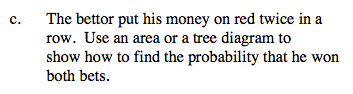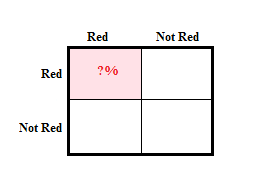### Home > A2C > Chapter 10 > Lesson 10.1.1 > Problem10-18

10-18.
1. A Nevada roulette wheel has 38 slots numbered 00, 0, 1, 2, 3, …, 36. Eighteen of the numbers 1, 2, …, 36 are red and 18 are black; 0 and 00 are green. Homework Help ✎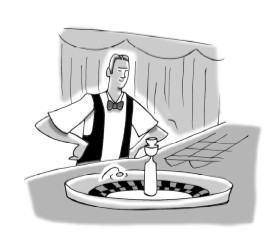1. What is the probability of landing on red?

2. What is the probability of not landing on red?

3. The bettor put his money on red twice in a row. Use an area or a tree diagram to show how to find the probability that he won both bets.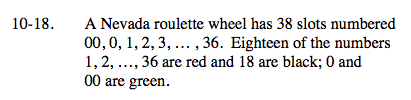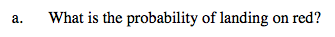$\frac{9}{4}\approx 47.4$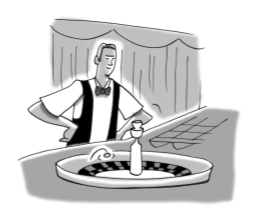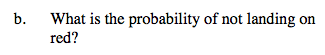$\frac{10}{19}=52.6$